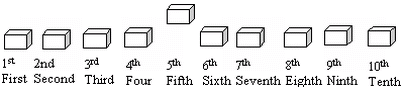Email us to get an instant 20% discount on highly effective K-12 Math & English kwizNET Programs!

Online Quiz (WorksheetABCD)

Questions Per Quiz = 2 4 6 8 10

Grade 1 - Mathematics2.25 Numbers and Number Sense - Review Test

 Q 1: 8, 9, 10, ___, 12Answer: Q 2: Count the number of stars and write down the number: * *Answer: Q 3: If you need one-half dozen eggs to bake a cake, how many eggs do you need?61231 Q 4: Count the number of items plus one more and write the answer.Answer: Q 5: Fill in the blank with greater than >, less than < or equal to =<>= Q 6: Count the number of stars and write down the number: *** ***Answer: Q 7: Here are 10 boxes. One of them is out of line. Name which one.5th2nd4th Q 8: 654EvenOdd Question 9: This question is available to subscribers only! Question 10: This question is available to subscribers only!